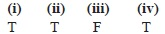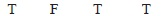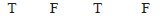# User ForumSubject :IMO    Class : Class 6

State 'T' for true and 'F' for false and select the correct option.
(i) A prime number cannot have 0, 2, 4, 5, 6 and 8 at its ones place except 2.
(ii) Two numbers are called co-primes, if their L.C.M. is 1.
(iii) If X is a factor of Y and Y is a factor of Z, then X is also a factor of Z.
(iv) 4 is the smallest composite number.

ABCDI think correct answer is B. Because all the numbers having 2 at ones are not prime number

Class : Class 6

## Ans 2:

Class : Class 9
Its not possible to have a number divisible by 2 in case of the digit at ones place being 5. So, (i) is false.(ii)F(iii)T(iv)TSo, B should be the answer. But the answer says C. Have no ideas why.

Class : Class 7
So (i) is false.

Class : Class 9

## Ans 5:

Class : Class 6
but 5 is primeso 1 is false

Class : Class 7

## Ans 7:

Class : Class 7
To be more specific, the statement should have been: -A prime number cannot have 0,2,4,5,6 and 8 at its ones place except for 2 and 5.

Class : Class 7
But 5 is prime

## Ans 9:

Class : Class 4
5 is prime so ( i ) is false but they have given that (i)is true why do they confuse us so much

## Ans 10:

Class : Class 6
ans is b 5 is already a prime number

## Ans 11:

Class : Class 6
5 will be in units place so it has to be option B

## Ans 12:

Class : Class 6
ans. is wrong ,ans. is B

## Ans 13:

Class : Class 9
5 is a prime number which makes the correct option as B

## Ans 14:

Class : Class 6
but 5 is prime so ( i ) is false but they have given that (i)is true

## Ans 15:

Class : Class 7
B is correct as 5 is a prime number in units place. Pls correct this Admin.

## Ans 16:

Class : Class 3
Ans is wrong. Opn B is correct.

## Ans 17:

Class : Class 6
5 itself is a prime number, so option i) cannot be true.The correct answer should be B.